Now that we understand light and lenses, we will be learning about how to quantify the effect that lenses have on light. This is the concept of lens power and it will put us well on our way to understand vision and glasses work.

To understand the concepts covered in this lesson, it is best to have read:

Now let’s begin!

## Lens Power

### What is Lens Power?

The power of a lens is also often referred to as the strength of a lens. It refers to how much of an effect a lens has on light passing through it.

A lens with high power has a big effect on the path of light passing through it and a lens with low power has a small effect.

### What Determines Lens Power?

The main determinant of lens power is the curvature of the lens.

The more curved a lens’ surface is, the more power it has and the more it will change the direction of light.

Insert Image

### What Types of Lens Powers Are There?

Recall from Lesson 2 that lenses can either be converging (+) or diverging (-).

1. Converging lenses have converging or plus power
2. Diverging lenses have diverging or minus power

## Plus Power

The following diagram will illustrate the concept of plus power.

It shows 3 different converging lenses, each with a different curvature.

The most curved lens has the most power and changes the direction of light the most.

The least curved (flattest) lens has the least power and changes the direction of light the least.## Minus Power

The next diagram will illustrate the concept of minus power.

It shows 3 different diverging lenses, each with a different curvature.

Again, the most curved lens has the most power and changes the direction of light the most.

The least curved (flattest) lens has the least power and changes the direction of light the least.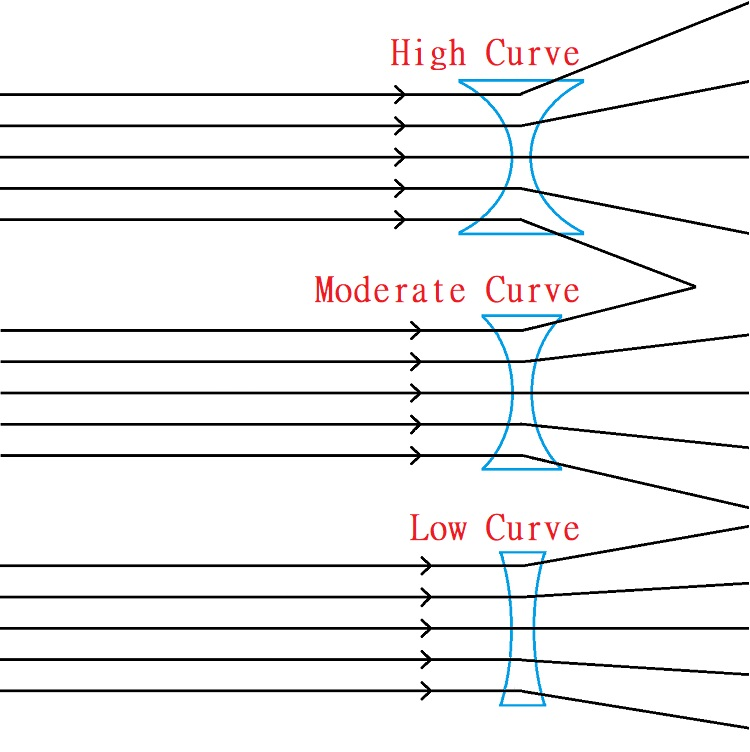Can you see that in both diagrams, the most curved lens ‘bends’ the light rays the most and the least curved lens ‘bends’ them the least? That is the result of each lens having a different power/strength, which is based on the curvature of the lens.

Now, in the real world, we need a way of describing the exact curvatures of lenses. We can’t just say ‘high curve’, ‘moderate curve’, or ‘low curve’ to describe lenses. That is not accurate enough.

Next, we will learn exactly how lens power is measured and quantified.

## How is Lens Power Measured?

It has to do with something called the focal distance of the lens. Let’s learn more about focal distance before we delve into lens power.

### Focal Distance of a Plus Lens

Recall from Lesson 1 that converging light eventually forms something called a focal point.

We’ve also learned from Lesson 2 that plus lenses cause incoming parallel light to become converging.

So if plus lenses cause light to converge and converging light forms a focal point, then every plus lens will create a focal point.

Let’s re-examine the following diagram. This time, note how the focal point for each lens is formed at different distances from the lenses themselves.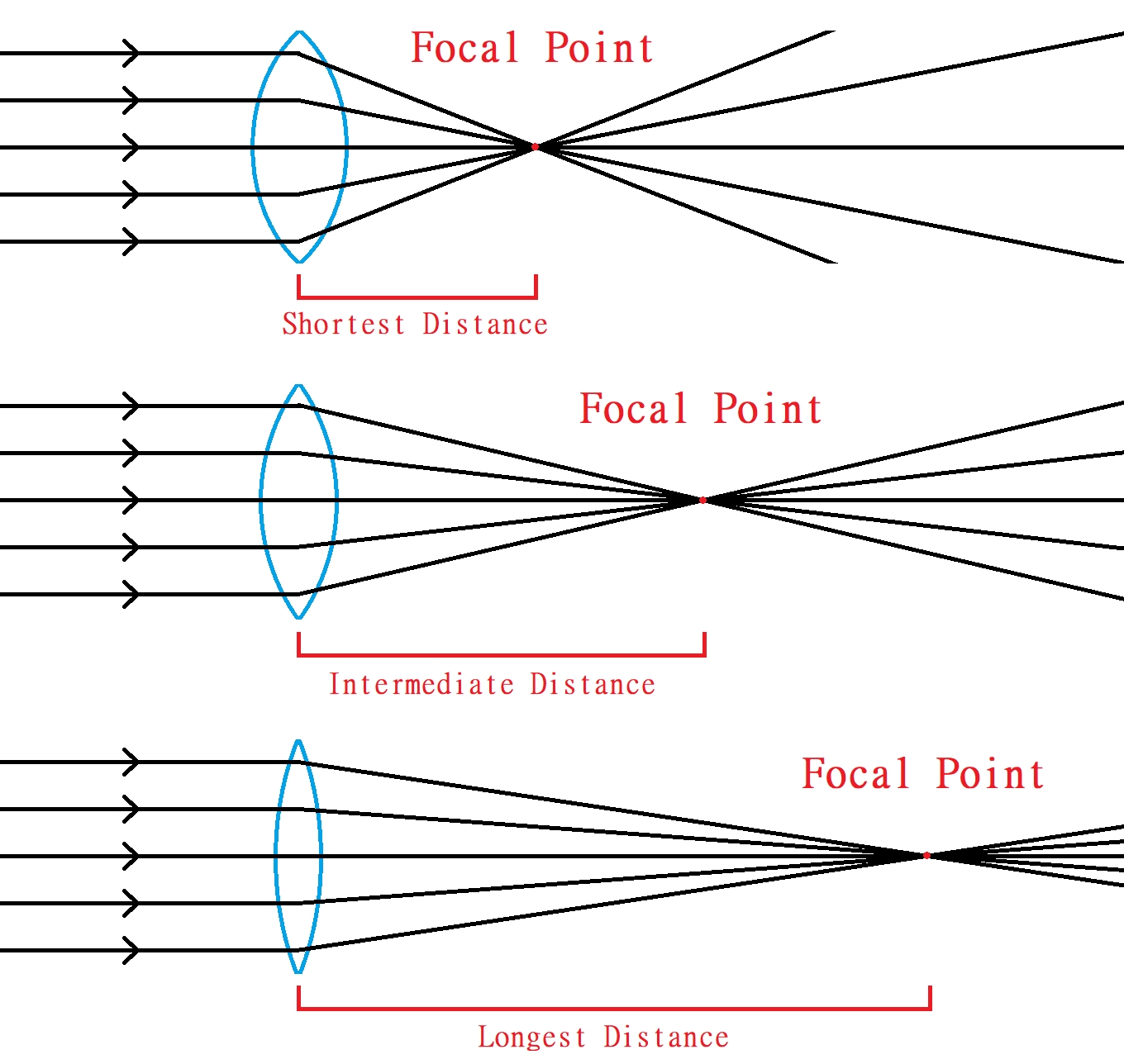The distance from the lens to the focal point is called the focal distance.

### Focal Distance of a Minus Lens

Just like plus lenses, minus lenses also form focal points. There’s just a small difference.

Remember that minus lenses cause light to diverge, meaning that once the light passes through a minus lens, the light rays move further and further apart from each other.

Now at this point, you may be wondering… If a focal point happens when all the light rays come together, then how can diverging light ever come to a focal point? That’s a great question!

In fact, the focal point doesn’t happen after the light passes through the lens, it happens before.

To find where the focal point is on a minus lens, you have to extend each of the light rays exiting the lens backward until they all meet.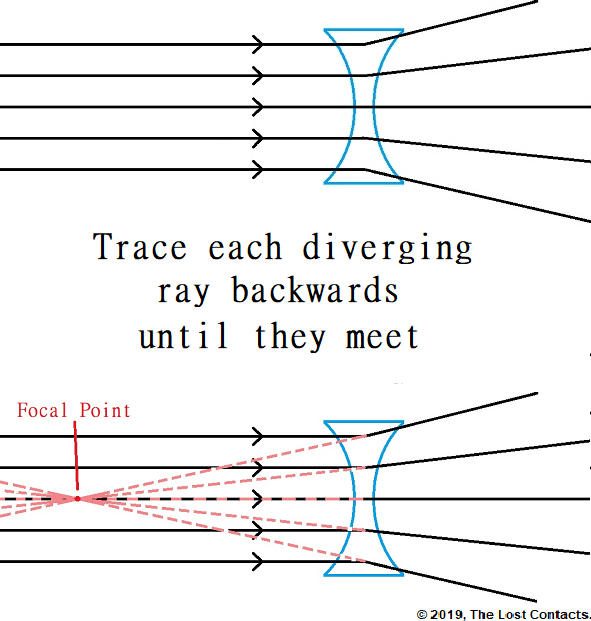We can do this with any minus lens. Let’s see what it looks like with our 3 minus lenses from earlier. Also note here that distance between the focal point and the lens increases as the lens gets flatter, just as it did with plus lenses.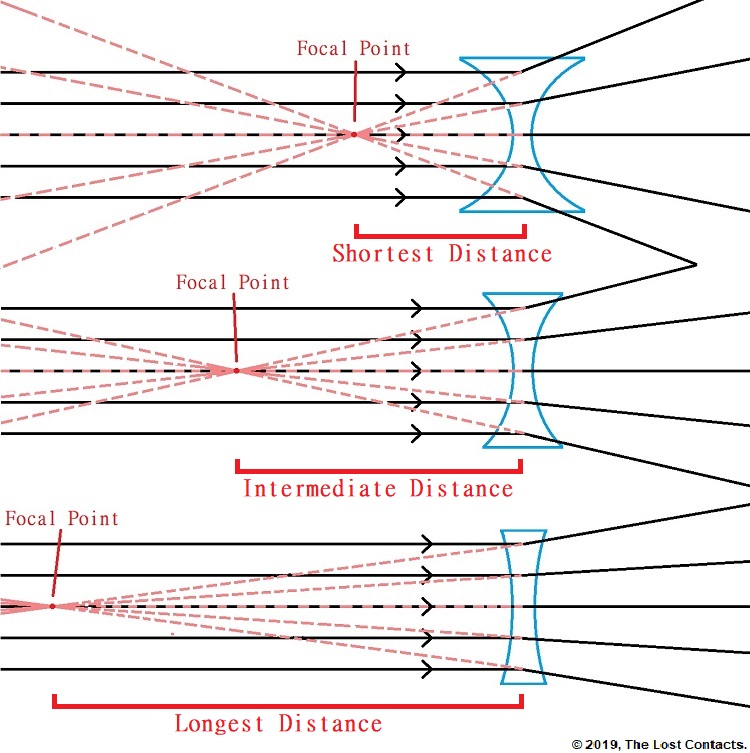Again, just as with plus lenses, the distance between the focal point and the lens is called the focal distance.

## Calculating Lens Power From Focal Distance

The power of any lens is based on its focal distance. To be more precise, the power of a plus lens is the inverse of its focal distance.

#### The power of a lens is the inverse of its focal distance.

What does this mean? Don’t worry, it’s not difficult!

In mathematical terms, the inverse of ‘something’ means dividing 1 by that ‘something’.

In this case, I said that the power of a plus lens is the inverse of its focal distance. The inverse of the focal distance means 1 divided by the focal distance. Therefore, lens power = 1 / focal distance.

#### Lens Power = 1 / Focal Distance

See, simple right?

Now there’s just one last thing to remember. When calculating lens power, we must use meters when measuring the focal distance. This produces a unit called Diopters, and that’s what lens power is measured in!

This will make a lot of sense when we start looking at examples.

We’re going to use the same diagrams as before but now we will assign some values to the focal distance so we can calculate the lens power.

### Calculating Plus Lens Power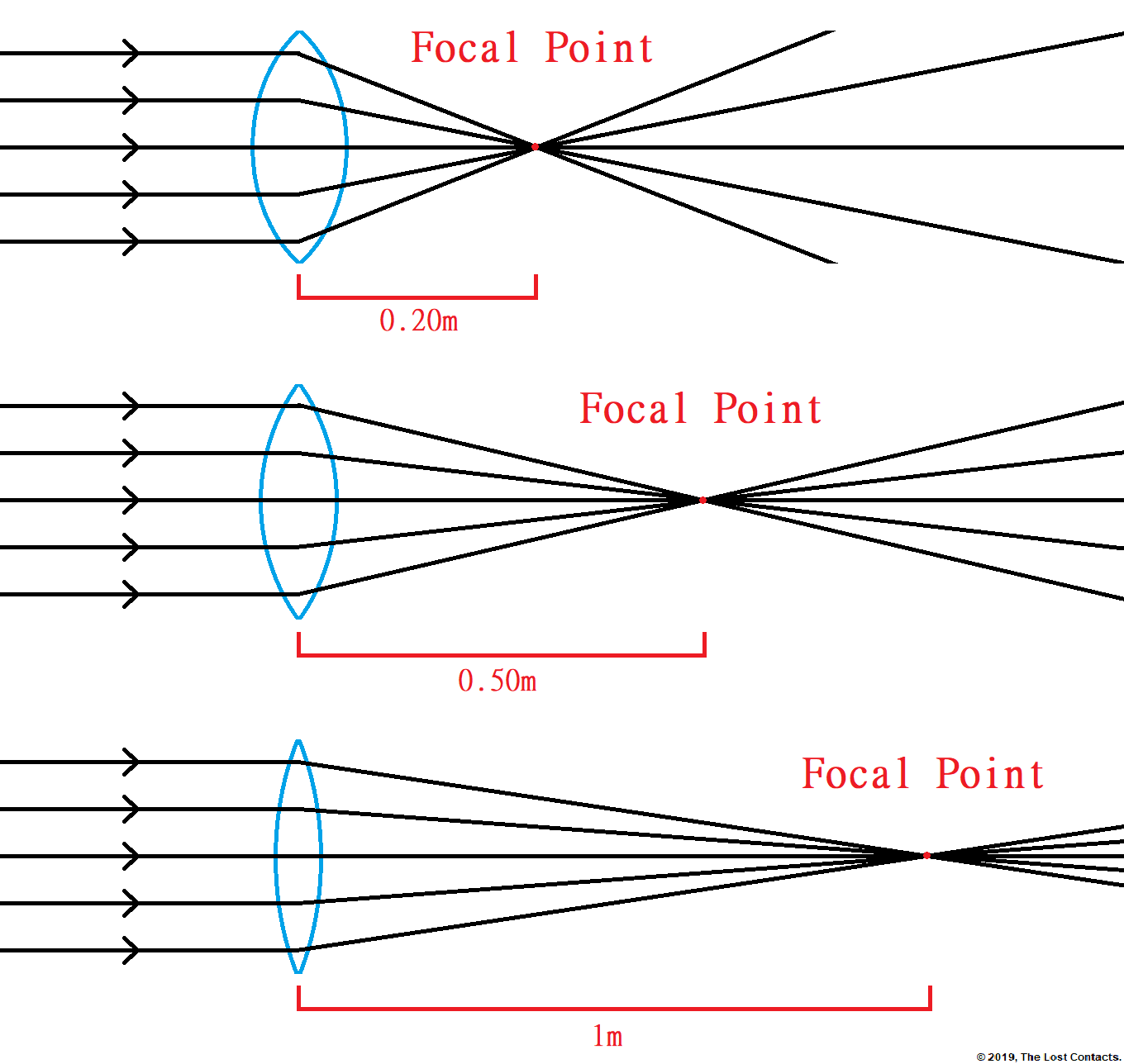Let’s calculate the lens power of each lens in the diagram.

### Top Lens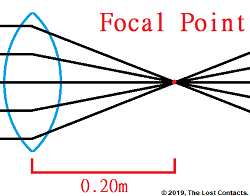The focal length is 0.20m.

The equation we’re using is:

Lens Power = 1 / Focal Length

The Calculation is:

Lens Power = 1 / 0.20m = +5D

### Middle LensThe focal length is 0.50m.

The equation we’re using is:

Lens Power = 1 / Focal Length

The Calculation is:

Lens Power = 1 / 0.50m = +2D

### Bottom Lens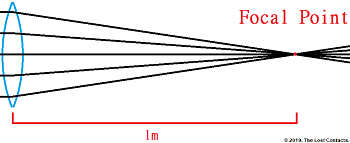The focal length is 1m.

The equation we’re using is:

Lens Power = 1 / Focal Length

The Calculation is:

Lens Power = 1 / 1m = +1D

That was easy right?

Let me just point out 2 things before we move onto minus lenses.

1. No conversions were necessary because all values were already in meters. If I had given the values 20cm, 50cm and 100cm, we would have had to convert them to meters before we did the calculations.
2. Because we were calculating the power of a plus lens, by convention we must include a plus sign in front of the lens power.

### Calculating Minus Lens Power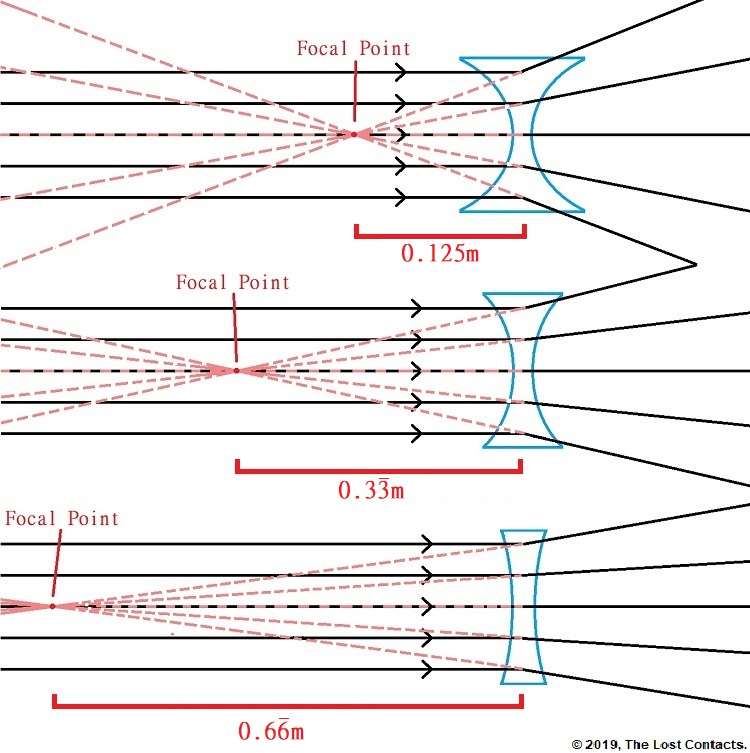Calculating the power of minus lenses may seem more complicated, but it’s the exact same steps.

### Top Lens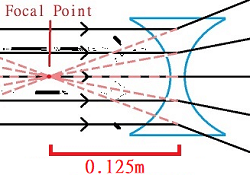The focal length is 0.125m.

The equation we’re using is:

Lens Power = 1 / Focal Length

The Calculation is:

Lens Power = 1 / 0.125m = -8D

###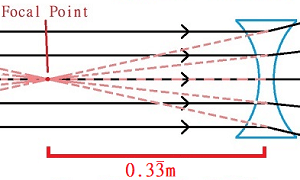The focal length is 0.333m.

The equation we’re using is:

Lens Power = 1 / Focal Length

The Calculation is:

Lens Power = 1 / 0.333m = -3D

### Bottom LensThe focal length is 0.666m.

The equation we’re using is:

Lens Power = 1 / Focal Length

The Calculation is:

Lens Power = 1 / 0.666m = -1.5D

Again, keep in mind these 2 things when calculating lens power.

1. No conversions were necessary for the examples above because all values were already in meters. If I had given the values 12.5cm, 33.3cm and 66.6cm, we would have had to convert them to meters before we did the calculations.
2. Because we were calculating the power of minus lenses, by convention we must include a minus sign in front of the lens power.

#### Key Points to Remember:

• Every lens (plus or minus) has a focal point
• The distance between the focal point and the lens is called the focal distance
• The formula for lens power is the same for plus or minus lenses:

Lens Power = 1 / Focal Distance

• The power of plus lenses are always denoted with a + (plus sign)
• The power of minus lenses are always denoted with a – (plus sign)

This lesson may take a few reads to really set in. If you still have questions about the material, feel free to leave them in the comments section and I will post some additional clarifications for you.

Great job making it this far into the course! This may be a good time to review Lessons 1,2 & 3 because in Lesson 4 we will switch gears and start learning about eye anatomy!